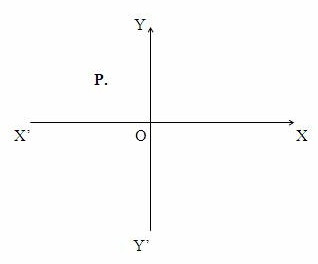## Sunday, January 06, 2013

### Questions (MCQ) Involving Gravitation

"Men often become what they believe themselves to be. If I believe I cannot do something, it makes me incapable of doing it. But when I believe I can, then I acquire the ability to do it even if I didn't have it in the beginning."
– Mahatma Gandhi

Today we shall discuss a few multiple choice questions involving gravitation. The first question pertains to a simple binary star system.

In a simple binary star system two stars orbit around their centre of mass under their mutual gravitational attractive force. The two stars constituting the binary star system need not be of the same mass. Note that Kepler’s laws are applicable to binary stars also. Click to see this.

Star systems with more than two stars (multiple star systems) are also present. About half of the stars in our galaxy, the milky way, are part of binary star systems or multiple star systems. Here is the first question:

(1) Consider a simple binary star system (Fig.) in which the member stars are identical, orbiting in a circle of radius r. If each star has mass m, the orbital speed of each star is
(a) √(Gm/r)
(b) √(Gm/2r)
(c) √(Gm/4r)
(d) √(2Gm/r)
(e) √(4Gm/r)
The gravitational force between the stars supplies the centripetal force required for the circular motion. Therefore we have
Gmm/(2r)2 = mv2/r where G is the gravitational constant and v is the orbital speed.
The above equation gives
v = √(Gm/4r)
The following question is related to a simple multiple star system:(2) Consider a multiple star system containing four stars of the same mass m arranged symmetrically and orbiting around their centre of mass C (Fig.) in the clockwise direction in a circle of radius r. What is the orbital speed of each star?

(a) 4[(Gm/r)(1+2√2)]1/2

(b) 2[(Gm/2r)(1+2√2)]1/2

(c) [(Gm/r)(1+2√2)]1/2

(d) (½)[(Gm/r)(1 + 2√2)]1/2

(e) (¼)[(Gm/r)(1+2√2)]1/2

The gravitational force F1 on a star due to the diametrically opposite star is given by

F1 = Gmm/(2r)2 = Gm2/4r2

[Note that the distance between the diametrically opposite stars is 2r]

Evidently F1 is directed towards the centre of mass C of the star system.

The other two stars exert gravitational forces F2 and F3 as shown in the figure. These forces are directed at 45º with respect to F1 and they have the same magnitude given by

F2= F3 = Gmm/(r√2)2 = Gm2/2r2

[Note that the distance between adjacent stars is (√2)r]

F2 and F3 have equal components (Gm2/2r2)cos 45º directed towards the centre of mass C of the star system. The net radial force acting on a star is therefore equal to F1 + F2 cos 45º + F3 cos 45º = Gm2/4r2 + (Gm2/2r2)cos 45º + (Gm2/2r2)cos 45º.

The radial force thus works out to (Gm2/r2)[(1/4) + (1/√2)] = (Gm2/r2)[(1 + 2√2)/4]

Since the above radial force supplies the centripetal force required for circular motion, we have

mv2/r =  (Gm2/r2)[(1 + 2√2)/4]

This gives the orbital speed, v = (½)[(Gm/r)(1 + 2√2)]1/2, as given in option (d).(3) A particle of mass 4m is kept fixed at point P (Fig.) in the xy-plane. Another free particle of mass m at the origin is found to be unperturbed when a third fixed particle of mass 6m also is present in the xy-plane. If the x-coordinate and y-coordinate of the particle of mass 4m are √2 and +√2 respectively, what are the x-coordinate and y-coordinate respectively of the particle of mass 6m?

(a) +√3 and √3

(b) √3 and +√3

(c) √6 and +√6

(d) +√2 and √2

(e) +3 and –3

The free particle of mass m at the origin is unperturbed since the gravitational forces on it due to the fixed particles are equal in magnitude and opposite in direction. This can happen only if the three particles are on the same straight line, with the free particle of mass m in between the fixed particles.

Equating the magnitudes of the gravitational forces, we have

G(4m)m/22 = G(6m)m/r2 where G is the constant of gravitation and r is the distance of the particle of mass 6m from the origin.

[Note that the distance of the particle of mass 6m from thr origin is {(√2)2 + (√2)2}1/2 = 2

The above equation gives

Gm2 = 6Gm2/r2 from which r = √6

Since the line joining the particles evidently bisects the angle XOY’ the x-coordinate and y-coordinate of the particle of mass 6m must be of equal value d such that

√(d2 + d2) = √6

Therefore d =√3

Since the particle of mass 6m is located in the 4th quadrant, its x-coordinate and y-coordinate are +√3 and √3 respectively [Option (a)].